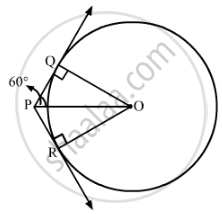# If from an external point P of a circle with centre O, two tangents PQ and PR are drawn such that ∠QPR = 120°, prove that 2PQ = PO. - Mathematics

If from an external point P of a circle with centre O, two tangents PQ and PR are drawn such that ∠QPR = 120°, prove that 2PQ = PO.

#### Solution

Let us draw the circle with external point P and two tangents PQ and PR.We know that the radius is perpendicular to the tangent at the point of contact.

∴∠OQP=90°

We also know that the tangents drawn to a circle from an external point are equally inclined to the segment, joining the centre to that point.

∴∠QPO=60°

Now in ∆QPO:

cos60^@="PQ"/"PO"

⇒1/2="PQ"/"PO"

⇒2PQ=PO

Concept: Number of Tangents from a Point on a Circle
Is there an error in this question or solution?